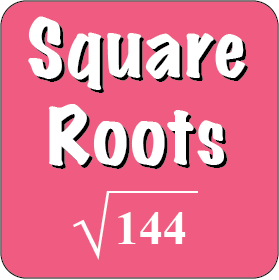Back to Mr. Anker TestsSquare Roots 1Name basic square roots and compare values with square roots.Square Roots 2Slightly more difficult square roots and comparisons with square roots.Square Roots 3More difficult questions and comparions with basic square roots.<Square Roots 4The square root of a number is between which two whole numbers?Square Roots 5The square root of a number is between which two whole numbers?Square Roots 6Using strategies to estimate a square root of a non-perfect square.Square Roots 7Using a procedure to break down a square root problem for a non-perfect square.Square Roots 8Using a procedure to break down a square root problem for a non-perfect square.Square Roots 9Using a procedure to break down a square root problem for a non-perfect square.